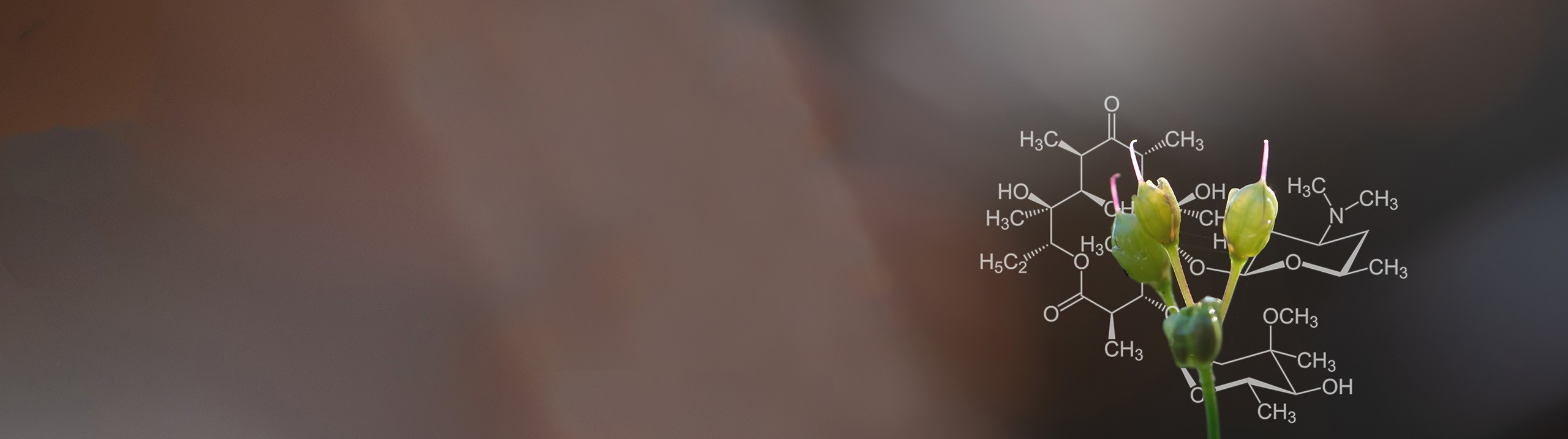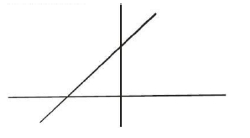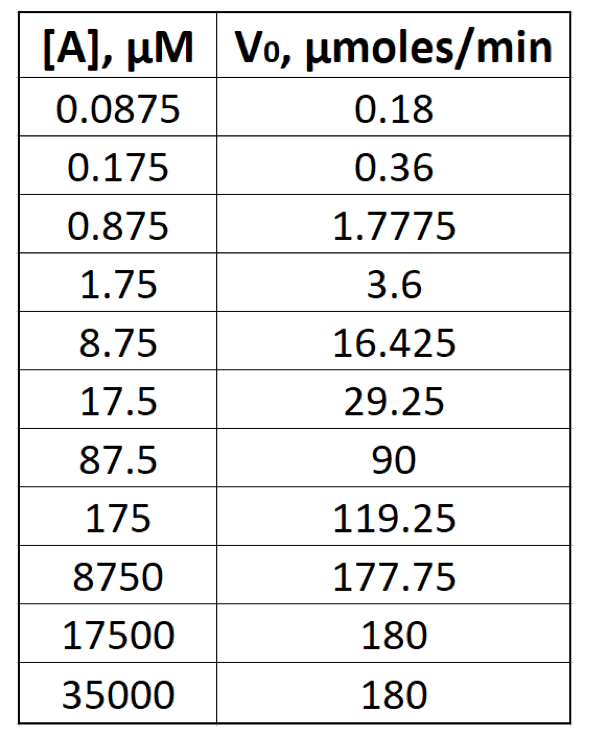Start typing, then use the up and down arrows to select an option from the list.# Biochemistry

Learn the toughest concepts covered in Biochemistry with step-by-step video tutorials and practice problems by world-class tutors

6. Enzymes and Enzyme Kinetics

# Lineweaver-Burk Plot

1

#### concept

Lineweaver-Burk Plot6m
Play a video:
2

#### concept

Lineweaver-Burk Plot8m
Play a video:
3

#### concept

Lineweaver-Burk Plot4m
Play a video:
all right. So now that we've introduced both the line Weaver, Burke equation and the line Weaver Burke plot in this lesson video, we're going to focus specifically on the why in the X intercepts of a line on a line Weaver Burke plot. Now the reason that we want to focus so much attention on the why and the Ex intercepts is because the most important information on a line we were Burke plot is actually revealed through these intercepts of the line. And that's because the why intercept in the X intercept are capable of revealing the V Max and the K M of an enzyme. But hang on. Not so fast, because the intercepts do not directly reveal the V Max in the K M instead. Since ah line Weaver Burke plot is also known as a double reciprocal plot. Pretty much everything is the reciprocal, including the intercepts. And so this means that the why intercept is going to reveal the reciprocal of the V Max. Essentially, the Y intercept is going to be one over the V max. And so if we want to get the V max from the Y intercept, then we need to take the reciprocal of the Y intercept now. Similarly, the X intercept is also going to be the reciprocal. Instead, the reciprocal is going to be of the K M and not of the V Max. Now we also need to recall from our previous lesson video that the X intercept on a line with Herbert plot will always fall into the negative region of the X axis. Which means that the X intercept is always gonna have a negative value and that it's always going to be the negative reciprocal of the K M. Which is why we have negative one over the K M. Now. Also recall from your previous math courses and chemistry courses and things like that that the why intercept is going to be where the line intercepts or crosses the Why access and that can Onley happen when the value of X is equal to zero. And, of course, the X intercept is going to be exactly where the line intercepts or crosses the X axis. And that can only happen when the value of why is equal to zero. And so if we take a look up at our line, we have Robert plot from our previous lesson. Videos recall that the Y axis is going to be the vertical axis here, and the X axis is going to be the horizontal axis. Now, the why intercept shown here is going to be exactly where our line crosses the why axis the vertical y axis, which is right at this point right here. Now, one way that helps me distinguish between the why and the ex intercepts. And what they reveal is that I know that on an enzyme kinetics plot, whether it be, um, Achilles meant an enzyme kinetics plot or a line with Herbert plot. This y axis I know is always associated with a reaction velocity. And so it's no surprise that the why intercept, which is associated with y Axis, is also related to a reaction velocity. More specifically, the maximum reaction velocity. And we know already that the Y intercept is going to be a reciprocal on a line with Robert plots. Since we know line with Robert, plots are also known as double reciprocal plots, and pretty much everything is the reciprocal, including thes intercepts. Now for similar reasoning, I know that the X intercept is always going to be associated with the X axis, which is where our line crosses the X axis, which is right at this position on this plot. And I know that because the X intercept is always associated with the X axis and the X axis, whether it be, um, Achilles meant in plot or a line with Herbert plot, it's always associated with a substrate concentration. And so I know that the X intercept, for that reason has to be associated with a substrate concentration, not of reaction velocity. And so recall that the K M is a substrate concentration, not a reaction velocity. And, of course, again, the X intercept we know is gonna be a reciprocal because line we've Robert plots are double reciprocal plots. So it's the reciprocal of the K M. And again recall that the X intercept always will fall into the negative region when it's a line Weaver Burke plot. And that means that the X intercept is actually going to be the negative reciprocal of the K M. And so moving forward will be able to get a lot more practice focusing on these Y and X intercepts in the information that they reveal. So that concludes this lesson, and I'll see you guys in our next video
4
Problem

To determine the Vmax from a Lineweaver-Burk plot you would:

5
Problem

A Lineweaver-Burk plot generates a line with the following formula: y = 0.3x + 0.4. What is the Km?

6
Problem

Consider the equation for the line on the following Lineweaver-Burk plot: y = 6x + 3A) What is the Km for the corresponding enzyme?

a) -2 mM

b) 18 mM

c) 2 mM

d) 9 mM

B) What is the Vmax for the corresponding enzyme?

a) 0.333 mM/s

b) 9 mM/s

c) -6 mM/s

d) 18 mM/s

7
Problem

Suppose the data on the right is plotted onto a Lineweaver-Burk plot to obtain a straight line.A) What is the value of the y-intercept of the line? ____________

B) What is the value of the x-intercept of the line? ____________